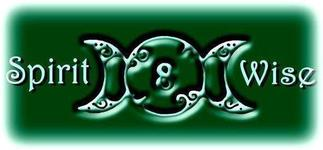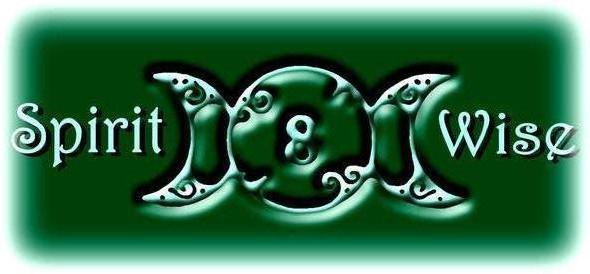## May 2018 Newsletter

 /* styles */ click on any title to read that section quickly
 /* styles */
 table div table+table+table+table+table+table div table{width:100%;padding:0}table div table+table+table+table+table+table div table img{width:96.23%;padding:0;float:none}table div table+table+table+table+table+table div table td{width:100%;padding:0 1.88% 18px}/* styles */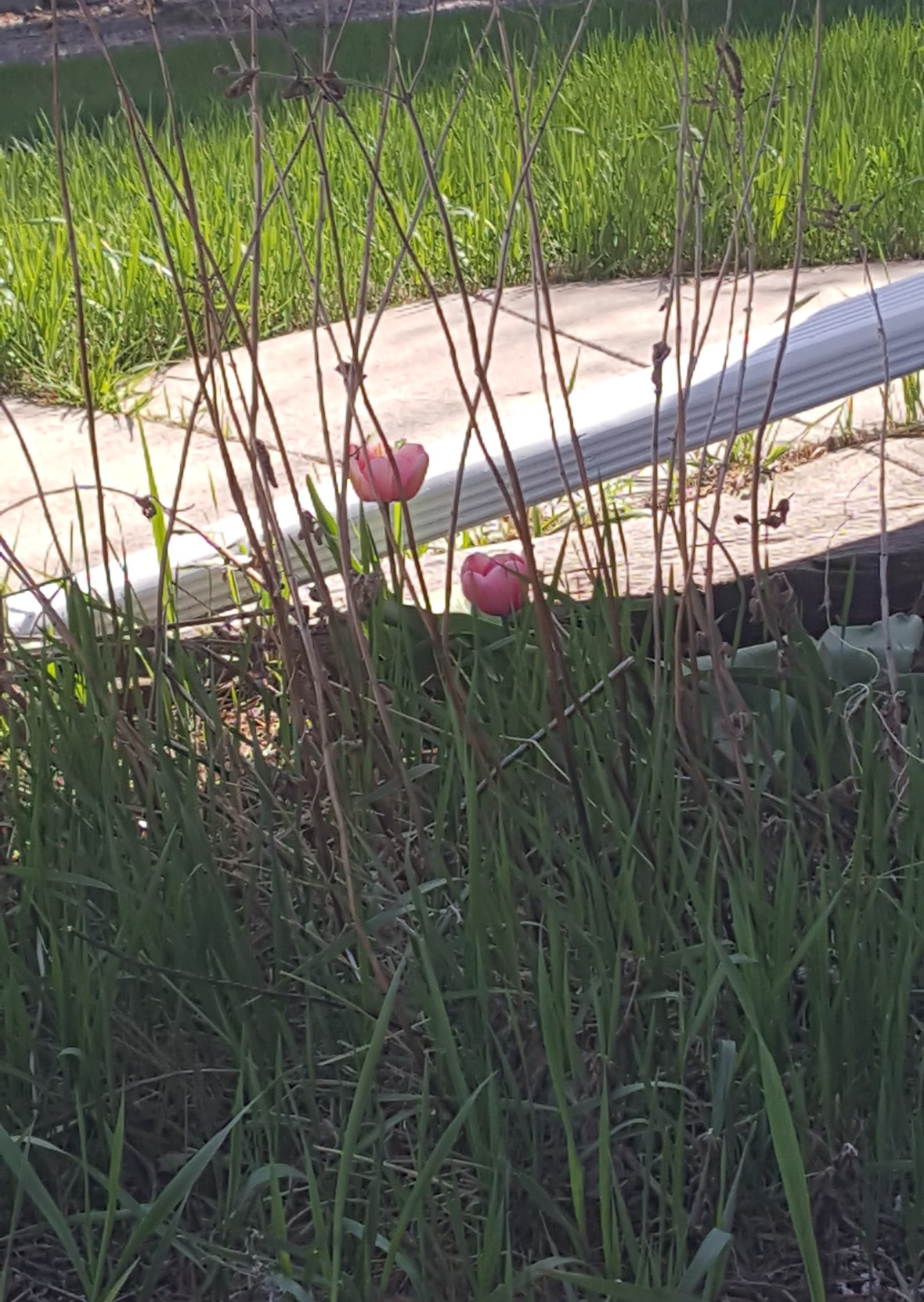Sometimes we have to look a little harder - to find Spring, to find beauty, to find something to celebrate. Sometimes it's just easier to see the worn and brambled life. It can be a lot of work, to focus on the bright moments.

And isn't that what May is for? To celebrate the life that wins through the Winter dark & the stormy Spring? Mothers work hard every day to create this life - and every day it blooms is a blessing! What better way to honor our Mothers - of the blood, of the spirit, of the Earth - than to take that moment and really see all the glorious new things that are pushing to the surface?

Every moment is a gift of new life; every breath an opportunity to see the beauty, to create the beauty, to honor the beauty. Every moment is the work of our own hands, and the hands of all who have come before.

All Hail, the Mothers of Creation. We are Beautiful.

 table div table+table+table+table+table+table+table+table div table{width:100%;padding:0}table div table+table+table+table+table+table+table+table div table img{width:96.23%;padding:0;float:none}table div table+table+table+table+table+table+table+table div table td{width:100%;padding:0 1.88% 18px}/* styles */## A Study in Stones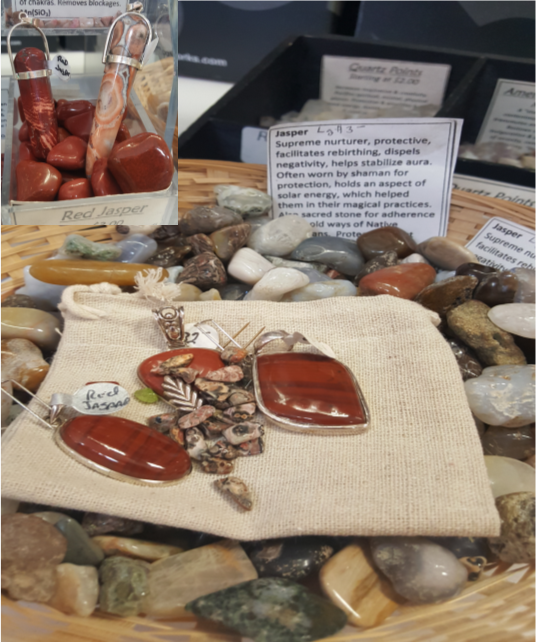Jasper comes in a variety of colors and names - red jasper, silverleaf jasper, brecciated jasper

There is also a Picture Jasper
Bat Cave Jasper
Wonderstone
And a store favorite - mookite.

On the flip side, jasper itself is a subcategory of chalcedony (however it's pronounced.)

# Jasper

Jasper is the nurturer - not only does it help ground self, balance the energies and smooth out the chakras, it can help keep one's energy higher during hospital stays and long fasts. And it helps the wearer to nurture and sustain others.

Jasper is "a stone which accepts great responsibility in the realm of protection." Are you picturing a mother bear? Yeah, me too. It helps eliminate negativity, smoothing out the auric energies.

It is very helpful in successful astral travel. And it seems to be highly connected to the sun.

Astro signs: Leo
Chakras: solar plexus
Body: internal organs
Vibration Numbers: 6
Type: mineral
Color: mostly reds, brown, and yellows, but also blue and green.

*information from Love is in the Earth

 /* styles */ Take me back to the Table of Contents!
 table div table+table+table+table+table+table+table+table+table+table+table+table+table div table{width:100%;padding:0}table div table+table+table+table+table+table+table+table+table+table+table+table+table div table img{width:96.23%;padding:0;float:none}table div table+table+table+table+table+table+table+table+table+table+table+table+table div table td{width:100%;padding:0 1.88% 18px}/* styles */## Monthly Special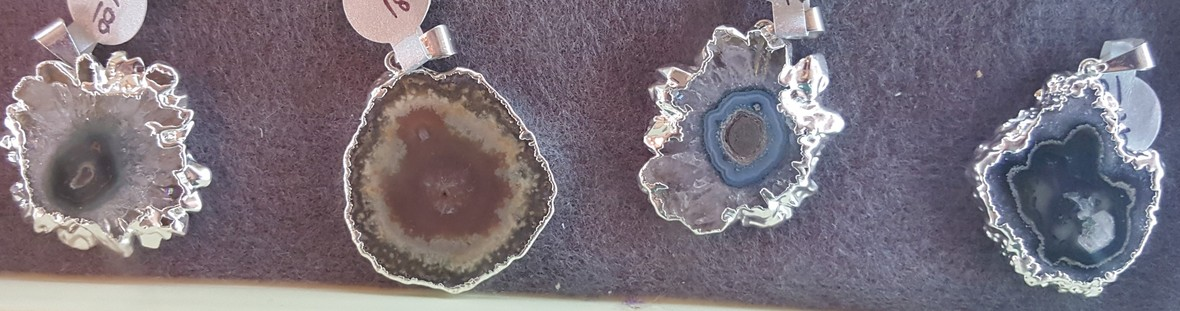# 20% off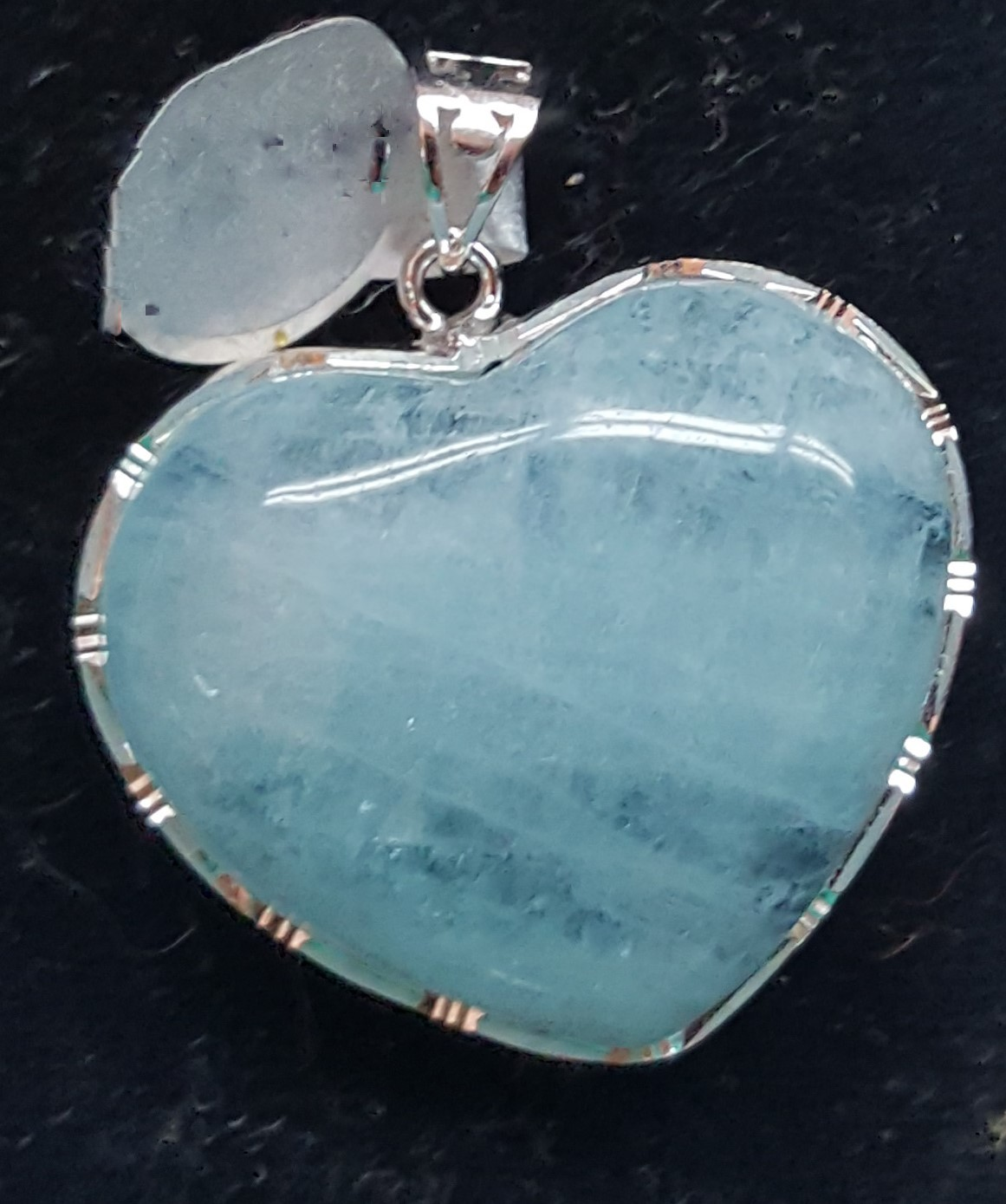## Sparkle, sparkle!

Shimmer, Shine

Mother Earth's treasures wait to be "present"ed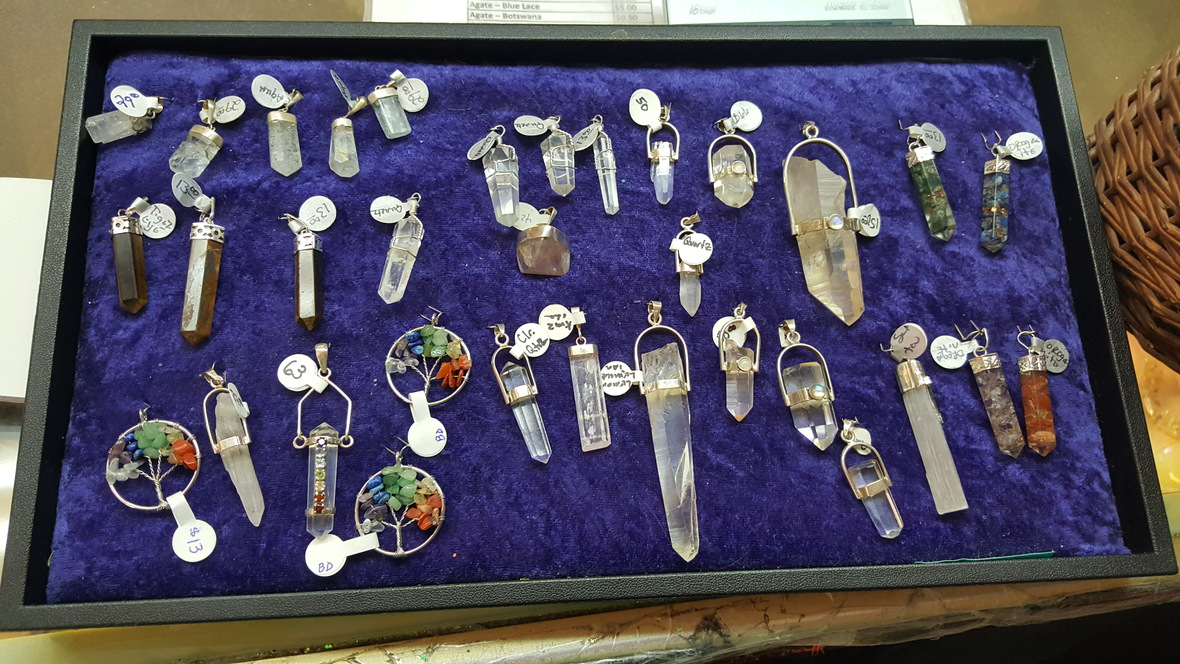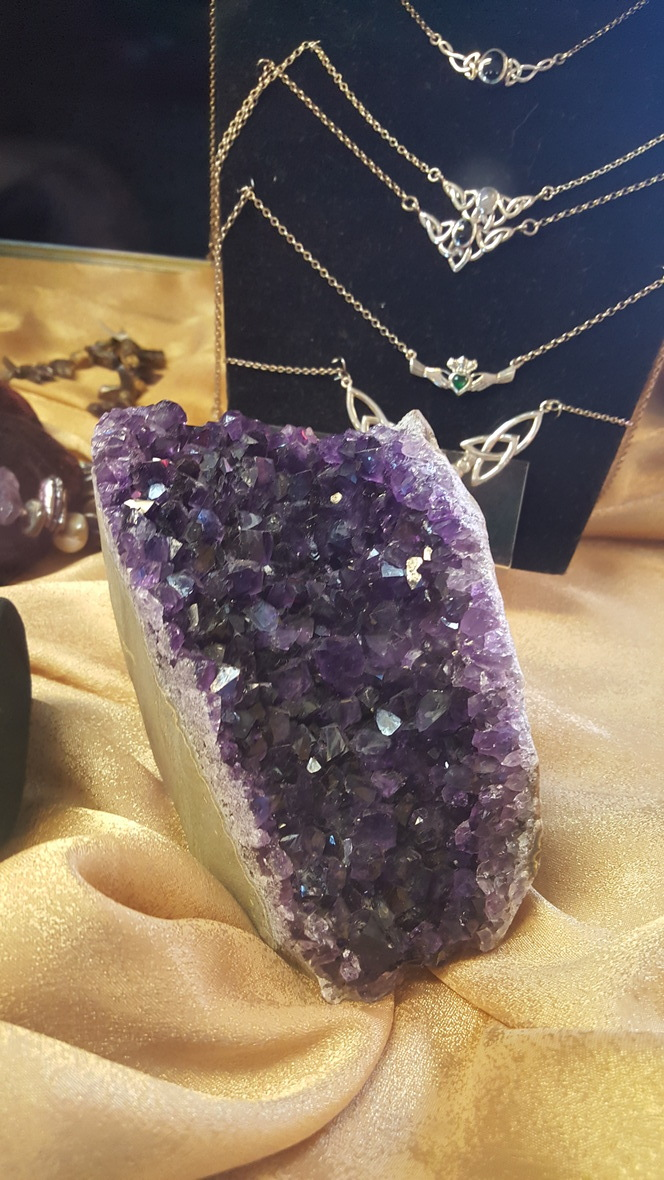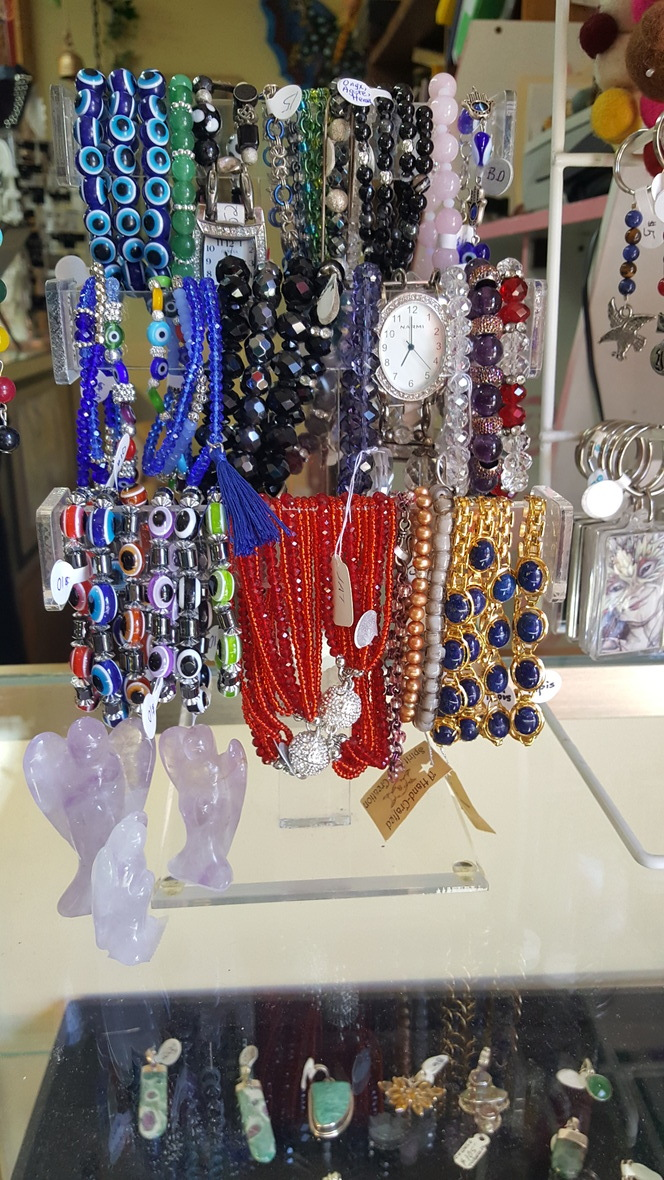/* styles */ Take me back to the Table of Contents!
 table div table+table+table+table+table+table+table+table+table+table+table+table+table+table+table+table+table+table+table div table{width:100%;padding:0}table div table+table+table+table+table+table+table+table+table+table+table+table+table+table+table+table+table+table+table div table img{width:96.23%;padding:0;float:none}table div table+table+table+table+table+table+table+table+table+table+table+table+table+table+table+table+table+table+table div table td{width:100%;padding:0 1.88% 18px}/* styles */## Connie Stahl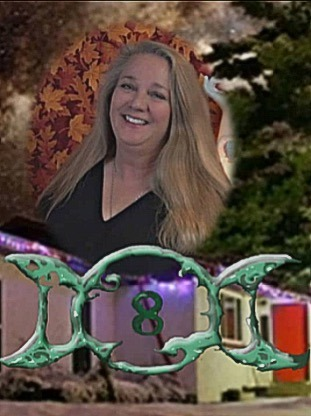# Premier Psychic

Connie Stahl, Spirit Wise' owner, is a hereditary clairvoyant and clairaudient reader, as well as High Priestess in her local coven.

Connie is currently available at Spirit Wise Thursday through Monday, from 1pm - 5pm. She is also available for private functions and private tarot tutoring.

 /* styles */ Take me back to the Table of Contents!

## Tim Christner# Brennan Healing

Tim Christner completed 4 years of training at the Barbara Brennan* School of healing, a world famous college in Miami with locations also in Europe and Japan.

Going beyond Reiki, this system of healing

can help to relieve pain, clear stagnant energy and past life debris, and give you back more of your “authentic” self.

The Human Energy Field contains 7 levels and 4 dimensions; the Physical, the Aura, the Hara (your level of intention) and the Core Star (your Divine Essence). "My education and innate ability allows me to bring all of these into balance for a total healing experience. This work can help you feel refreshed and more like yourself again!"

## Why not try a powerful Energy Healing?

To schedule a session, call Tim Christner at 720-254-0815 or email: tclighthealing@gmail.com (Rates are \$75.00 per hour)

Morning and evening appointments are available to meet your scheduling needs.

 /* styles */ Take me back to the Table of Contents!
 table div table+table+table+table+table+table+table+table+table+table+table+table+table+table+table+table+table+table+table+table+table+table+table+table+table+table div table{width:100%;padding:0}table div table+table+table+table+table+table+table+table+table+table+table+table+table+table+table+table+table+table+table+table+table+table+table+table+table+table div table img{width:96.23%;padding:0;float:none}table div table+table+table+table+table+table+table+table+table+table+table+table+table+table+table+table+table+table+table+table+table+table+table+table+table+table div table td{width:100%;padding:0 1.88% 18px}/* styles */## Other Services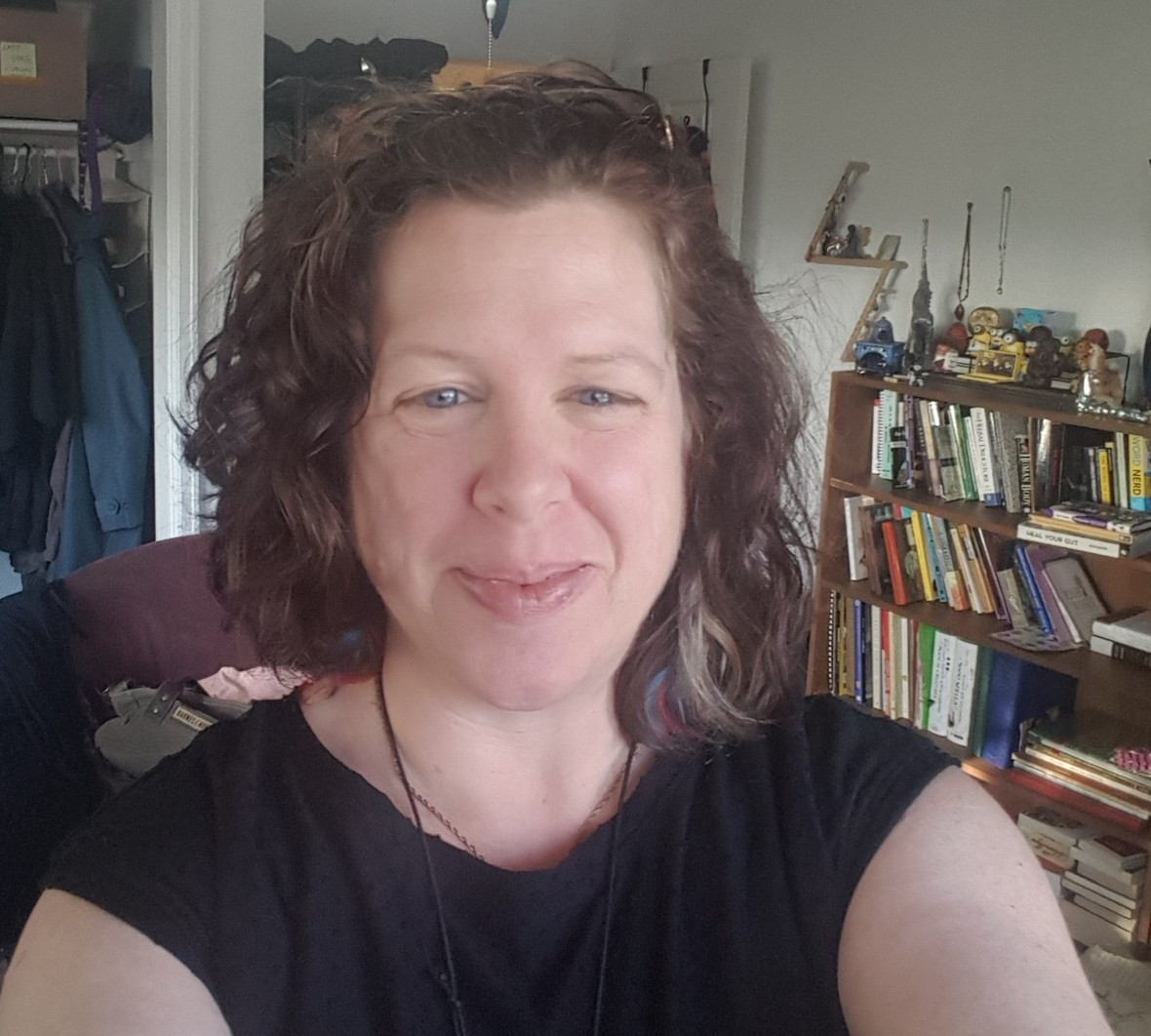## Lila Allen - Happiness Practitioner

I come from a family of huggers and positive thinkers. I am an empath, and I enjoy hanging out with the Elements, and translating the Universe through runes. A session could also include intuitive conversation, tarot, and magic planning. Happiness is my specialty - the unique, awesomely expansive happiness we are all destined for.

I am available on Tuesdays and by appointment. 303-408-6900 / lilaallen@unicallen.com

 /* styles */ Take me back to the Table of Contents!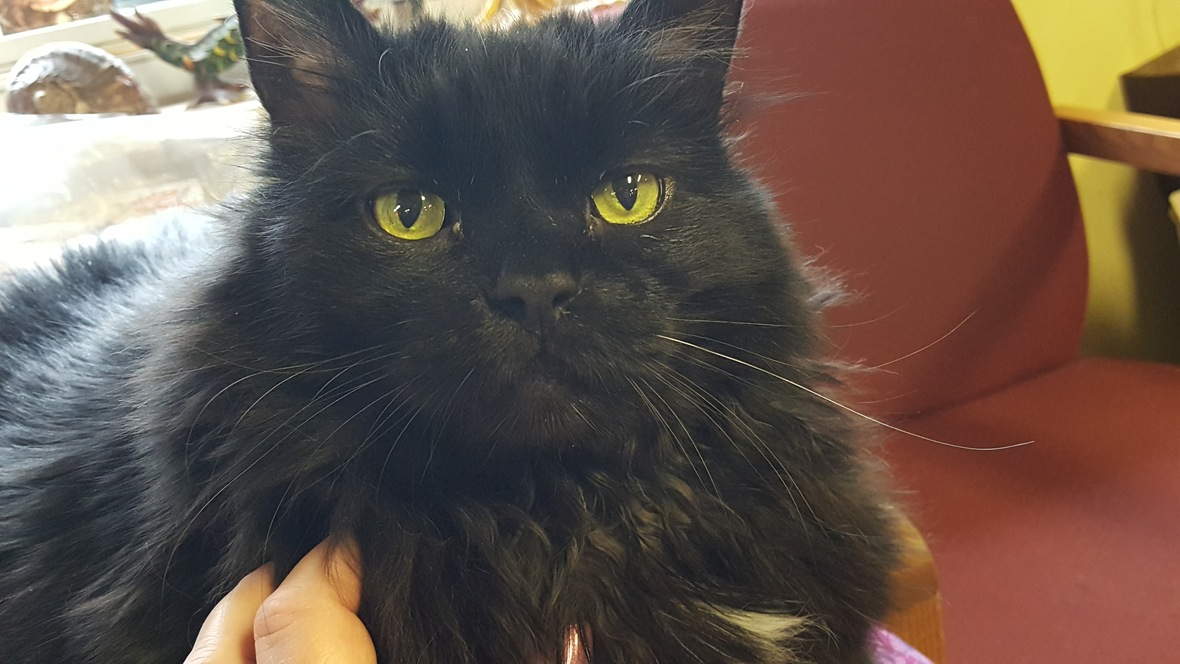## Sampson - Energy Work

Sampson is available for adoration during most business hours, and sometimes provides assistance in finding that perfect item. (When he feels like it, of course.)

And yes, he will root through your purse to learn all about you. If you are abuzz, he could help ground you. And of course, he can tell you all the secrets of the universe.

He won't. But he could.

 table div table+table+table+table+table+table+table+table+table+table+table+table+table+table+table+table+table+table+table+table+table+table+table+table+table+table+table+table+table+table+table div table{width:100%;padding:0}table div table+table+table+table+table+table+table+table+table+table+table+table+table+table+table+table+table+table+table+table+table+table+table+table+table+table+table+table+table+table+table div table img{width:96.23%;padding:0;float:none}table div table+table+table+table+table+table+table+table+table+table+table+table+table+table+table+table+table+table+table+table+table+table+table+table+table+table+table+table+table+table+table div table td{width:100%;padding:0 1.88% 18px}/* styles */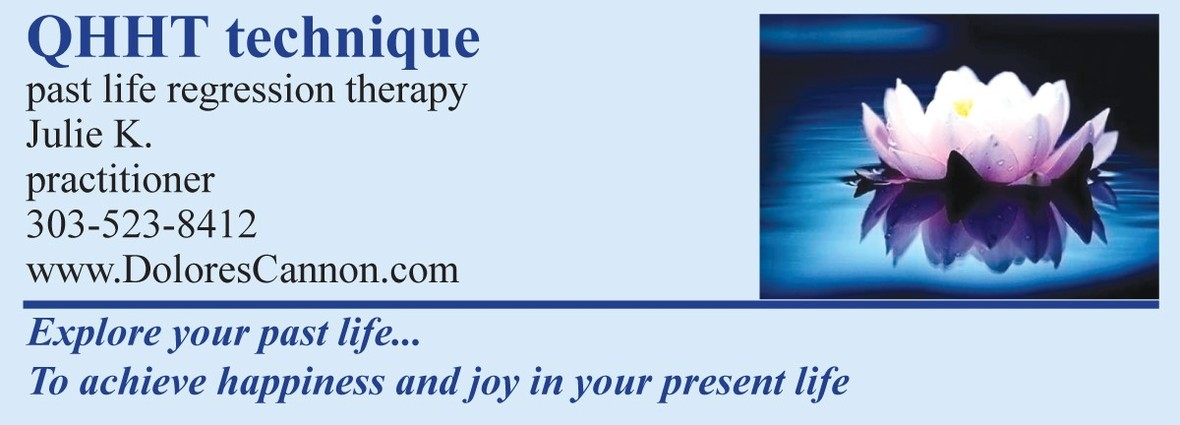## Julie K - Past Life Regression

▪ Wish you could have the answers to life's most important questions?
▪ Curious about Past Life Regression (PLR?)
▪ Want to experience hypnosis first-hand?
 ▪ Wish you could have the answers to life's most important questions?
 ▪ Curious about Past Life Regression (PLR?)
 ▪ Want to experience hypnosis first-hand?

Try the Quantum Healing Hypnosis Technique (QHHT), developed by renowned metaphysical author Dolores Cannon.

During a Quantum Healing Hypnosis session, the conscious mind is asked to step aside and observe, without interfering, while the Subconscious steps forward. The Subconscious acknowledges a problem, identifies its source, and provides ways to overcome it.

contact Julie K to set your appointment!

 /* styles */ Take me back to the Table of Contents!
 table div table+table+table+table+table+table+table+table+table+table+table+table+table+table+table+table+table+table+table+table+table+table+table+table+table+table+table+table+table+table+table+table+table+table div table{width:100%;padding:0}table div table+table+table+table+table+table+table+table+table+table+table+table+table+table+table+table+table+table+table+table+table+table+table+table+table+table+table+table+table+table+table+table+table+table div table img{width:96.23%;padding:0;float:none}table div table+table+table+table+table+table+table+table+table+table+table+table+table+table+table+table+table+table+table+table+table+table+table+table+table+table+table+table+table+table+table+table+table+table div table td{width:100%;padding:0 1.88% 18px}/* styles */## What's New?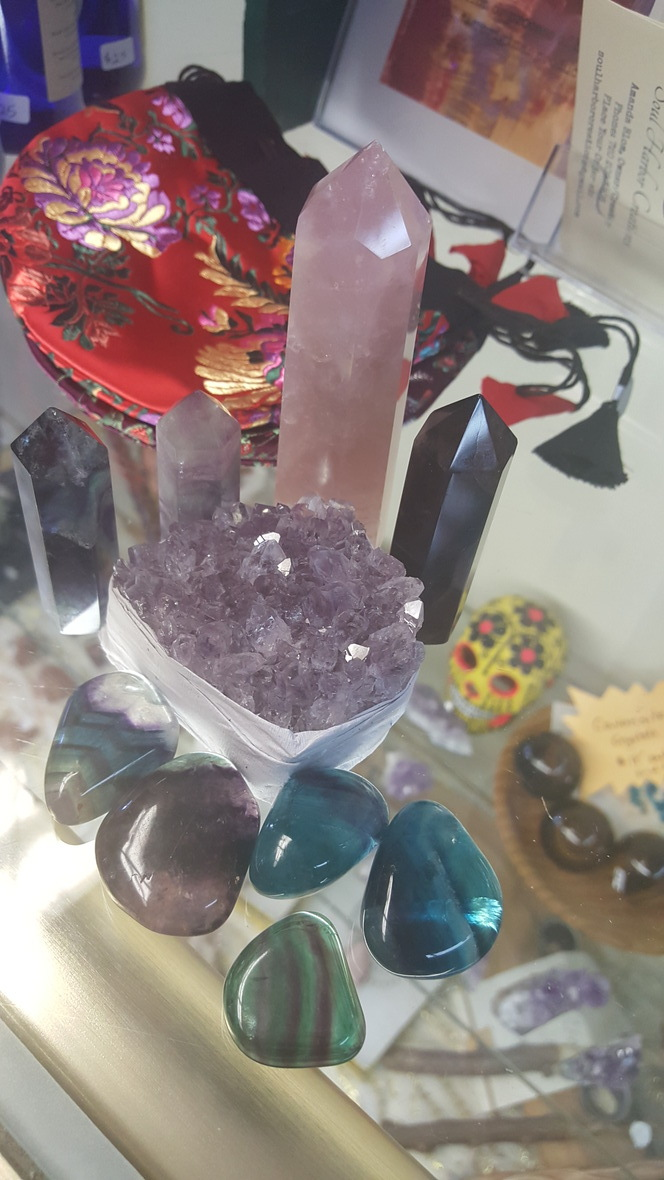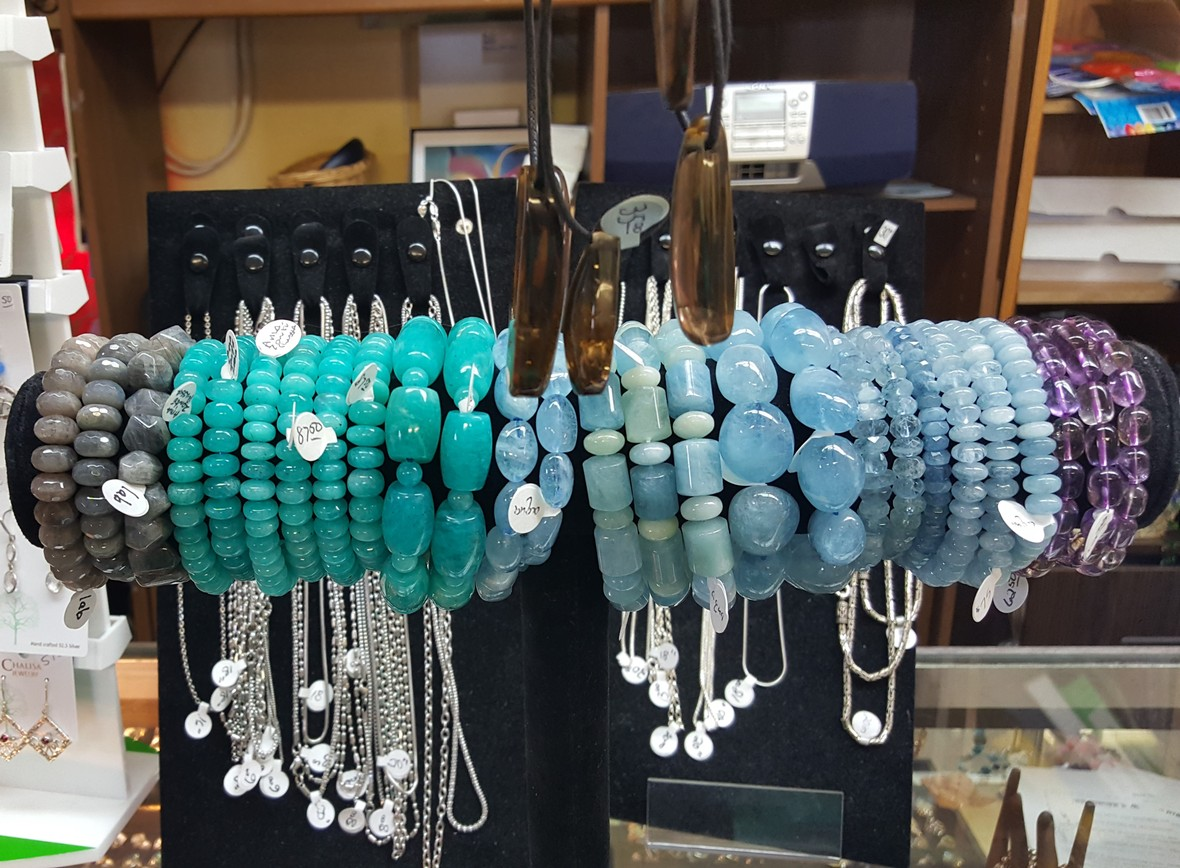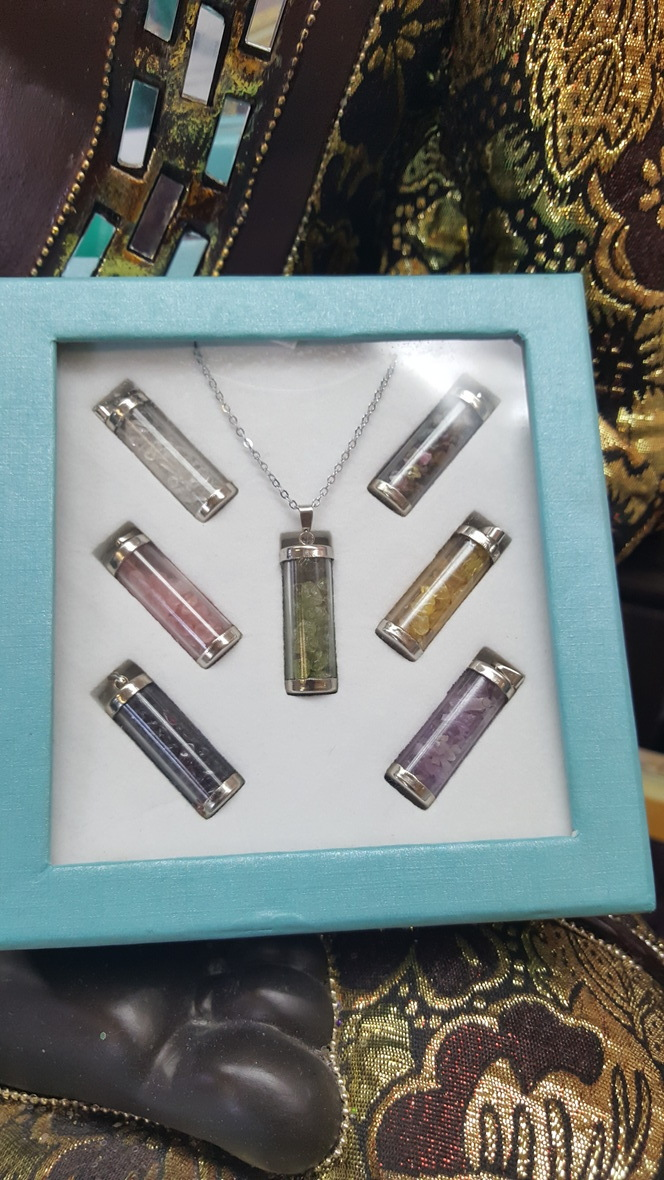/* styles */ Take me back to the Table of Contents!
 table div table+table+table+table+table+table+table+table+table+table+table+table+table+table+table+table+table+table+table+table+table+table+table+table+table+table+table+table+table+table+table+table+table+table+table+table+table+table div table{width:100%;padding:0}table div table+table+table+table+table+table+table+table+table+table+table+table+table+table+table+table+table+table+table+table+table+table+table+table+table+table+table+table+table+table+table+table+table+table+table+table+table+table div table img{width:96.23%;padding:0;float:none}table div table+table+table+table+table+table+table+table+table+table+table+table+table+table+table+table+table+table+table+table+table+table+table+table+table+table+table+table+table+table+table+table+table+table+table+table+table+table div table td{width:100%;padding:0 1.88% 18px}/* styles */## Ogham ExplorationTree: Iubhar / Yew
Letter: I
Bird: Illiat / Eaglet
Color: Irfind / White

Did you know a new yew is born through the death of the old one? It grows within the remains of the dead tree - a phoenix of a tree, a natural example of transformation and the cycle of life.

Yet in order for that new life, the old one must pass. Transformation is about moving from one life to the next.

Sometimes it just happens; the storms sweep through and we are left with devestation and transformation and the strenth to survive both.

But sometimes the transformation is gentle, reticent, waiting for us to make that first step. If we can be as strong as the yew - resilient and full of belief; if we can KNOW that the end is the beginning, we can BECOME.

The message of the yew - be strong enough to be magic; be magic enough to let go; be free enough to transform into your true strength.

What is growing inside you?

I hope you have a great month,
-Lila

*information from Ogham, the Celtic Oracle, Peter Pracownik & Andy Baggott

 /* styles */ Take me back to the Table of Contents!
 table div table+table+table+table+table+table+table+table+table+table+table+table+table+table+table+table+table+table+table+table+table+table+table+table+table+table+table+table+table+table+table+table+table+table+table+table+table+table+table+table+table+table div table{width:100%;padding:0}table div table+table+table+table+table+table+table+table+table+table+table+table+table+table+table+table+table+table+table+table+table+table+table+table+table+table+table+table+table+table+table+table+table+table+table+table+table+table+table+table+table+table div table img{width:96.23%;padding:0;float:none}table div table+table+table+table+table+table+table+table+table+table+table+table+table+table+table+table+table+table+table+table+table+table+table+table+table+table+table+table+table+table+table+table+table+table+table+table+table+table+table+table+table+table div table td{width:100%;padding:0 1.88% 18px}/* styles */## May 1 BELTANE

May 5 (Sat): FERI DUST and the IRON PENTACLE 1:00 - 6:00 pm
Tues (starting May 8): YOGA CLASS : 5 - 6 pm
May 15 (Tues): New Moon - 5:49 am *
May 20 (Sun) : DIVINATION EXCHANGE: 12:30 - 2:30 pm
May 20 (Sun) : Sun enters Gemini - 8:15 pm +*
May 29 (Tues) : Full Moon - 8:21 am *

Please connect with us on FB or Twitter for updates on events, and store hours!

Most astrological information is from Colorado Celtic Weekly Planner (Paulie Rainbow). some info from cafeastrology.com

 table div table+table+table+table+table+table+table+table+table+table+table+table+table+table+table+table+table+table+table+table+table+table+table+table+table+table+table+table+table+table+table+table+table+table+table+table+table+table+table+table+table+table+table+table+table div table{width:100%;padding:0}table div table+table+table+table+table+table+table+table+table+table+table+table+table+table+table+table+table+table+table+table+table+table+table+table+table+table+table+table+table+table+table+table+table+table+table+table+table+table+table+table+table+table+table+table+table div table img{width:96.23%;padding:0;float:none}table div table+table+table+table+table+table+table+table+table+table+table+table+table+table+table+table+table+table+table+table+table+table+table+table+table+table+table+table+table+table+table+table+table+table+table+table+table+table+table+table+table+table+table+table+table div table td{width:100%;padding:0 1.88% 18px}/* styles */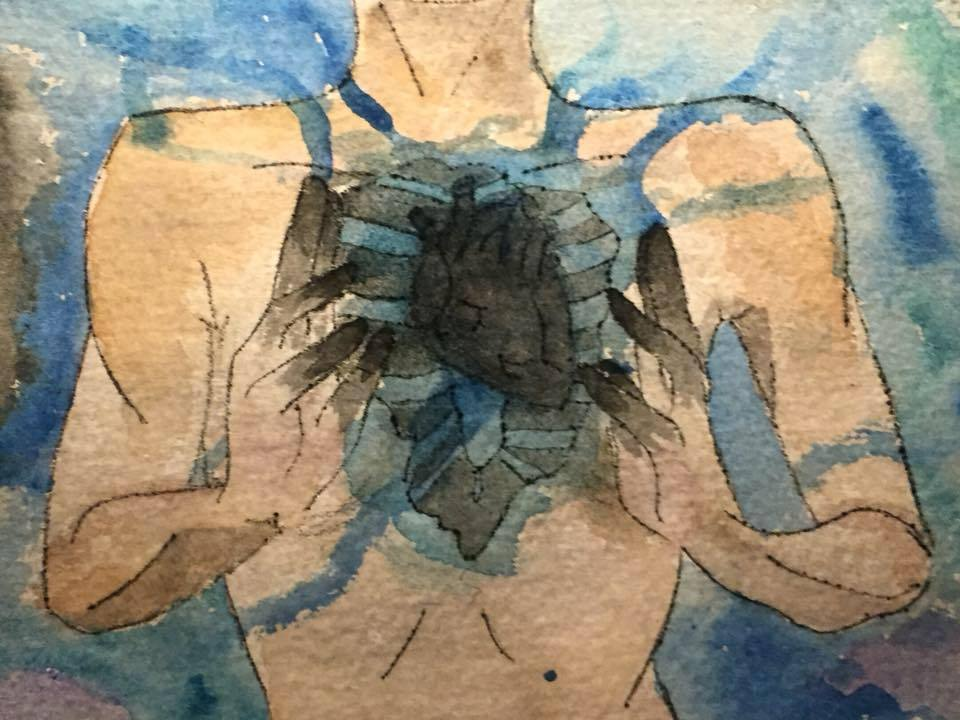# Feri Dust & the Iron Pentacle

## May 5th

This experiential workshop explores the ancient witchy powers of the Pentacle in its most distinctively human manifestation--the Pentacle of Iron. Our human lives and our magic are grounded in the energies of Sex, Self, Passion, Pride and Power. We will work with the tools and techniques of transformation and self-exploration within sacred ritual space. To this class, bring all of who you are, and all you hope to become.

Using our magical skills, moving and shaping energy, transforming ourselves through trance to explore the five points of our inner pentacle: Sex (primal energy), Self, Passion, Pride (self-esteem), and Power (effectiveness in the worlds). Sprinklings of Feri lore and mysticism inform this ecstatic work.

 /* styles */ Tuition will be adjusted according to attendance._ Saturday, May 5 1:00 – 6:00 Class (3rd of 3) 6:00pm Closing Ritual You may wish to bring a journal, a water bottle, drums or rattles, a divination tool such as runes or Tarot, a potluck lunch item to share, a compassionate heart and a mirthful and reverent mind. The Iron Pentacle will be drug and alcohol free so safe, nurturing, sacred space is created for all. Cost: \$55 to \$95 sliding scale Deposit: \$25.00 deposit is nonrefundable unless class canceled by the teachers. Deposit is applied toward tuition. The organizers believes strongly in making classes available to everyone in the community. Scholarship or work exchange is available Call: Skathach @ 303-618-7702 EMAIL: skathachsophia@gmail.com
 table div table+table+table+table+table+table+table+table+table+table+table+table+table+table+table+table+table+table+table+table+table+table+table+table+table+table+table+table+table+table+table+table+table+table+table+table+table+table+table+table+table+table+table+table+table+table+table+table div table{width:100%;padding:0}table div table+table+table+table+table+table+table+table+table+table+table+table+table+table+table+table+table+table+table+table+table+table+table+table+table+table+table+table+table+table+table+table+table+table+table+table+table+table+table+table+table+table+table+table+table+table+table+table div table img{width:96.23%;padding:0;float:none}table div table+table+table+table+table+table+table+table+table+table+table+table+table+table+table+table+table+table+table+table+table+table+table+table+table+table+table+table+table+table+table+table+table+table+table+table+table+table+table+table+table+table+table+table+table+table+table+table div table td{width:100%;padding:0 1.88% 18px}/* styles */# Yoga Classes

## Tuesdays 5pm - 6pm

Gentle, alignment based yoga classes include mindful movement with cues on body alignment. Students will build some heat but leave feeling relaxed and refreshed!

Lila can attest! "The pace was gentle, the movements a continuous flow, and I left feeling like I'd had a massage on the inside."

\$10 cash per class

## Walk Ins are Welcome! First Class is FREE

 table div table+table+table+table+table+table+table+table+table+table+table+table+table+table+table+table+table+table+table+table+table+table+table+table+table+table+table+table+table+table+table+table+table+table+table+table+table+table+table+table+table+table+table+table+table+table+table+table+table+table+table div table{width:100%;padding:0}table div table+table+table+table+table+table+table+table+table+table+table+table+table+table+table+table+table+table+table+table+table+table+table+table+table+table+table+table+table+table+table+table+table+table+table+table+table+table+table+table+table+table+table+table+table+table+table+table+table+table+table div table img{width:96.23%;padding:0;float:none}table div table+table+table+table+table+table+table+table+table+table+table+table+table+table+table+table+table+table+table+table+table+table+table+table+table+table+table+table+table+table+table+table+table+table+table+table+table+table+table+table+table+table+table+table+table+table+table+table+table+table+table div table td{width:100%;padding:0 1.88% 18px}/* styles */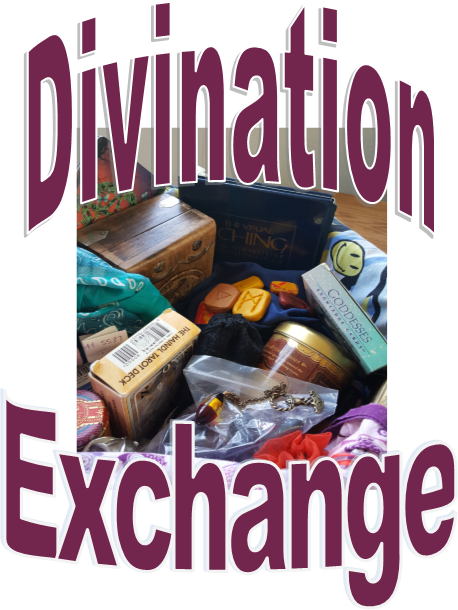# Divination Exchange

Sunday, May 20
12:30 - 2:30 pm

## Let's Play with the Future!

Come and exchange energy work with other practitioners. Reading usually last 15 - 30 minutes each, so please arrive by 2 to give and receive.

Any modality, any experience level.

## All Welcome

hosted by Lila

 /* styles */ Take me back to the Table of Contents!
 table div table+table+table+table+table+table+table+table+table+table+table+table+table+table+table+table+table+table+table+table+table+table+table+table+table+table+table+table+table+table+table+table+table+table+table+table+table+table+table+table+table+table+table+table+table+table+table+table+table+table+table+table+table+table div table{width:100%;padding:0}table div table+table+table+table+table+table+table+table+table+table+table+table+table+table+table+table+table+table+table+table+table+table+table+table+table+table+table+table+table+table+table+table+table+table+table+table+table+table+table+table+table+table+table+table+table+table+table+table+table+table+table+table+table+table div table img{width:96.23%;padding:0;float:none}table div table+table+table+table+table+table+table+table+table+table+table+table+table+table+table+table+table+table+table+table+table+table+table+table+table+table+table+table+table+table+table+table+table+table+table+table+table+table+table+table+table+table+table+table+table+table+table+table+table+table+table+table+table+table div table td{width:100%;padding:0 1.88% 18px}/* styles *//* styles */ Are you hosting an event at Spirit Wise? Make sure we all get the news - send the information to lilaallen@unicallen.com.
 table div table+table+table+table+table+table+table+table+table+table+table+table+table+table+table+table+table+table+table+table+table+table+table+table+table+table+table+table+table+table+table+table+table+table+table+table+table+table+table+table+table+table+table+table+table+table+table+table+table+table+table+table+table+table+table+table div table{width:100%;padding:0}table div table+table+table+table+table+table+table+table+table+table+table+table+table+table+table+table+table+table+table+table+table+table+table+table+table+table+table+table+table+table+table+table+table+table+table+table+table+table+table+table+table+table+table+table+table+table+table+table+table+table+table+table+table+table+table+table div table img{width:96.23%;padding:0;float:none}table div table+table+table+table+table+table+table+table+table+table+table+table+table+table+table+table+table+table+table+table+table+table+table+table+table+table+table+table+table+table+table+table+table+table+table+table+table+table+table+table+table+table+table+table+table+table+table+table+table+table+table+table+table+table+table+table div table td{width:100%;padding:0 1.88% 18px}/* styles */## Rare Tarot Decks Now Available!

 /* styles */ We have a collection of rare, out of print, and HIGHLY collectable cards. Because of their high value, these cards are not on display. All cards are in wonderful condition! Please call Connie at 303-790-2974 for more information or to view a specific deck.

We're getting new used books (ha ha) out every week.

We'll even take a look at yours!

## Books on magic and complete Divination decks always welcome!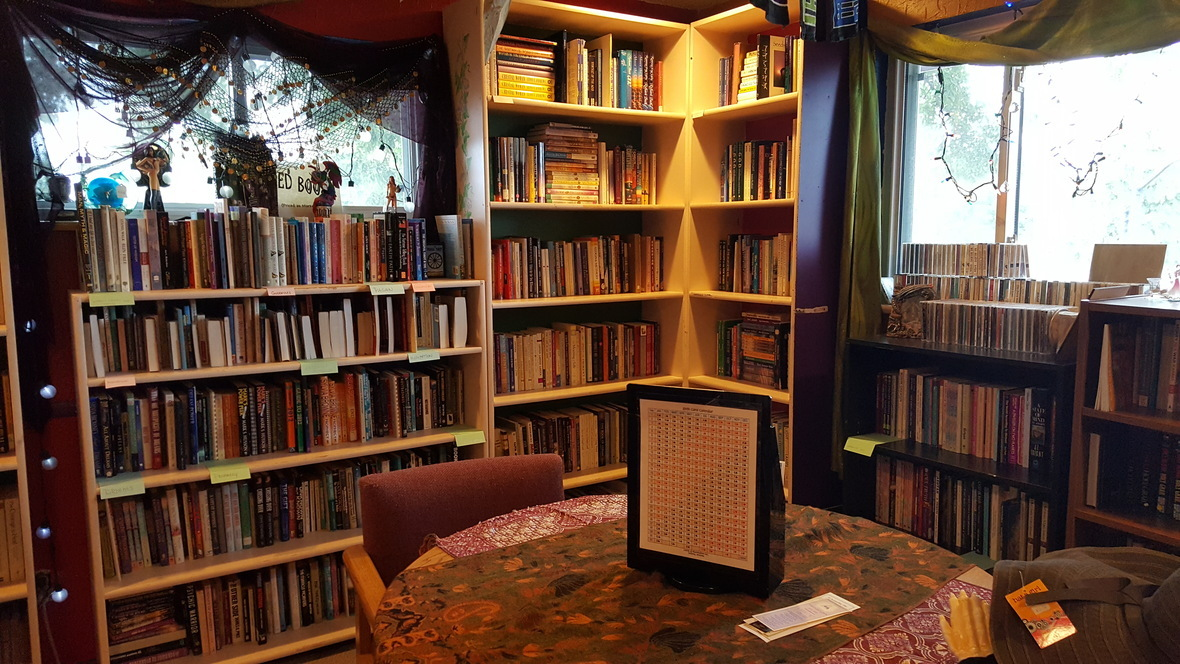/* styles */ Ask us about our rare and collectible used books, too!
 table div table+table+table+table+table+table+table+table+table+table+table+table+table+table+table+table+table+table+table+table+table+table+table+table+table+table+table+table+table+table+table+table+table+table+table+table+table+table+table+table+table+table+table+table+table+table+table+table+table+table+table+table+table+table+table+table+table+table+table+table+table+table div table{width:100%;padding:0}table div table+table+table+table+table+table+table+table+table+table+table+table+table+table+table+table+table+table+table+table+table+table+table+table+table+table+table+table+table+table+table+table+table+table+table+table+table+table+table+table+table+table+table+table+table+table+table+table+table+table+table+table+table+table+table+table+table+table+table+table+table+table div table img{width:96.23%;padding:0;float:none}table div table+table+table+table+table+table+table+table+table+table+table+table+table+table+table+table+table+table+table+table+table+table+table+table+table+table+table+table+table+table+table+table+table+table+table+table+table+table+table+table+table+table+table+table+table+table+table+table+table+table+table+table+table+table+table+table+table+table+table+table+table+table div table td{width:100%;padding:0 1.88% 18px}/* styles */## Event Space Available!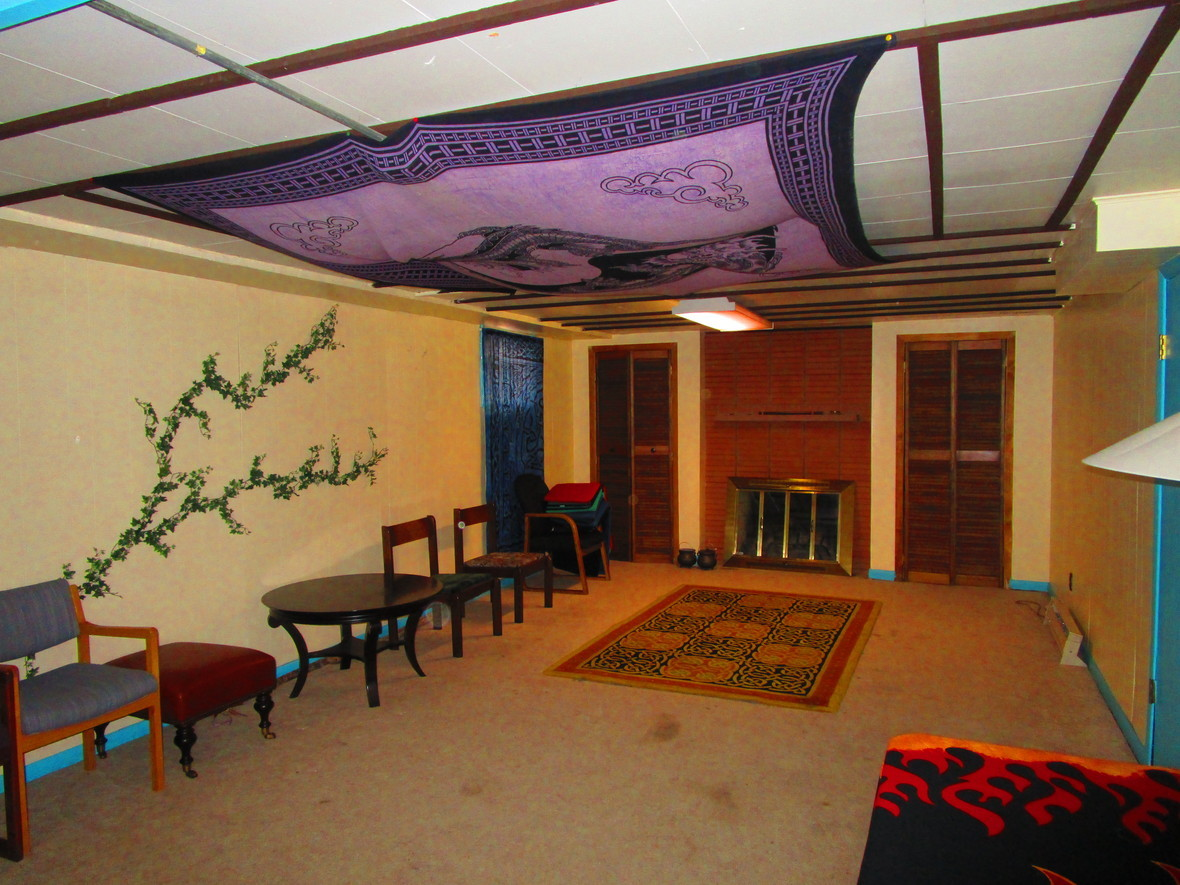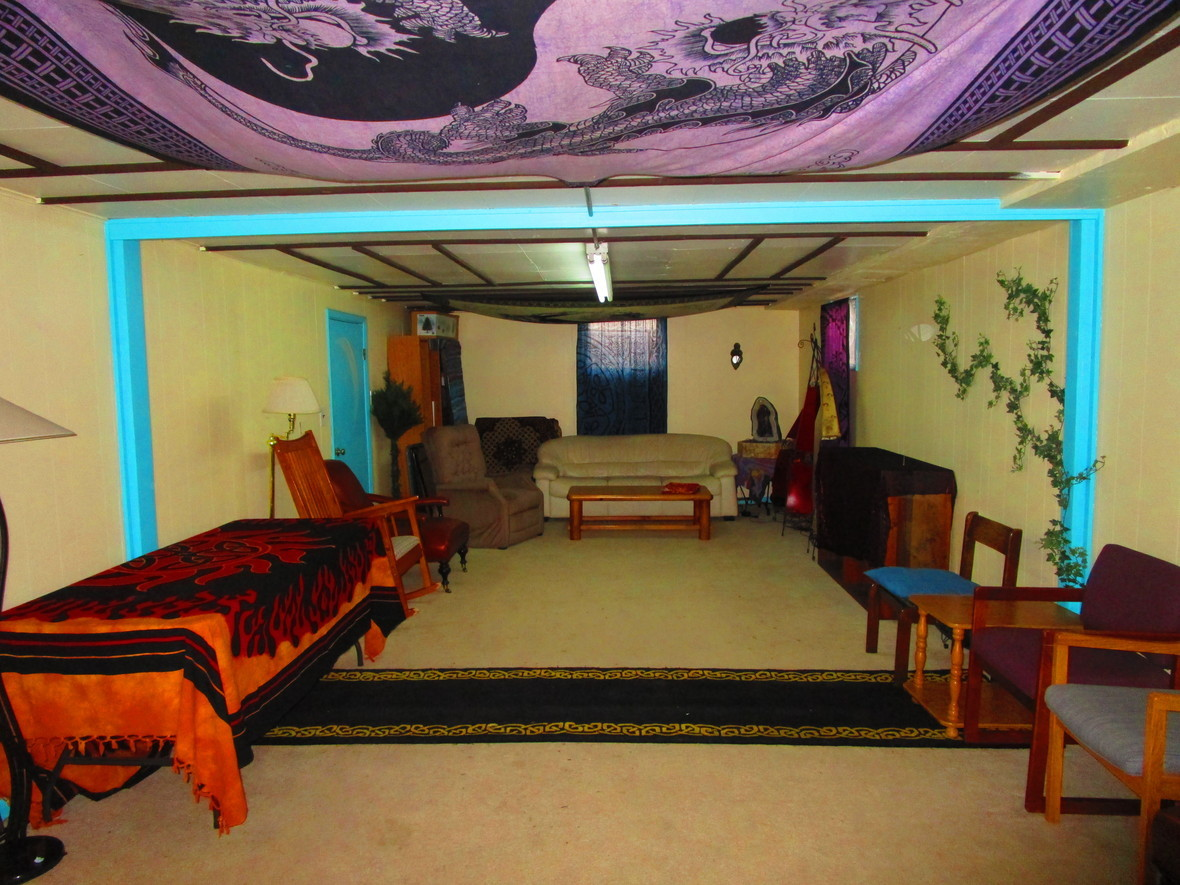/* styles */ Are you looking for a comforting place for your yoga class, drum circle, or workshop? Have the people but need the room? We have the perfect rental space for you. Clean and cool, this lovely space is available any time you need it - not just store hours - at an unbeatable price. We charge only a small percentage of your event earnings (or a \$30 minimum) regardless of the length of your event, and your price includes free advertising in our monthly newsletter. Contact us at 303-730-2974 to reserve your space today!
 /* styles */ Take me back to the Table of Contents!
 table div table+table+table+table+table+table+table+table+table+table+table+table+table+table+table+table+table+table+table+table+table+table+table+table+table+table+table+table+table+table+table+table+table+table+table+table+table+table+table+table+table+table+table+table+table+table+table+table+table+table+table+table+table+table+table+table+table+table+table+table+table+table+table+table+table+table+table div table{width:100%;padding:0}table div table+table+table+table+table+table+table+table+table+table+table+table+table+table+table+table+table+table+table+table+table+table+table+table+table+table+table+table+table+table+table+table+table+table+table+table+table+table+table+table+table+table+table+table+table+table+table+table+table+table+table+table+table+table+table+table+table+table+table+table+table+table+table+table+table+table+table div table img{width:96.23%;padding:0;float:none}table div table+table+table+table+table+table+table+table+table+table+table+table+table+table+table+table+table+table+table+table+table+table+table+table+table+table+table+table+table+table+table+table+table+table+table+table+table+table+table+table+table+table+table+table+table+table+table+table+table+table+table+table+table+table+table+table+table+table+table+table+table+table+table+table+table+table+table div table td{width:100%;padding:0 1.88% 18px}/* styles */Like   Tweet   Pin   +1